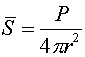Examples for electromagnetic waves

Example #1

Problem:

a.) If a radio station broadcasts at 88.9 MHz, what is the wavelength of the signal?

Solution:

Use l = c/f.

l = 3.37 m

b.) How long does it take sunlight to reach the earth? DATA: Rearth's orbit = 1.5 E8 km.

Solution:

The time is given by:t = R/c.

t = 500 s = 8 min., 20 sec.

Example #2

Problem:

A satellite emits a radio signal with an average power of 1000 W. The signal is spread evenly in all directions. What is the rms strength of the electric field from the radio wave, when one is three million km away.

Solution:

First, calculate the intensity of the light, with= 1.1 E-16 W/m2.

Next, calculate the Electric field strength using.

E = 2.89 E-7 V/m

Example #3

Problem:

a.) Light of an intensity of 100 W/m2, directed along the z axis and polarized along the x axis is incident is incident on a single polarizer oriented along the y axis. What is the intensity of the resulting y-plane polarized light.

Solution:

The cosine of 90 degrees is zero.

Save = 0 W/m2

b.) For the same problem as above, suppose one uses 2 polarizers, one oriented midway between the x and y axis while the second one is oriented along the y axis.

Solution:

The cosine of 45 degrees is 1/sqrt(2), so each polarizer cuts out half the intensity.

Save = 25 W/m2

c.) For the same problem as above, suppose one uses 90 ideal polarizers, each one oriented one degree past the preceeding one.

Solution:

The fraction that comes through will be cos( 1.0 degrees)180.

Save = 97 W/m2